# PI Function in ExcelClaudia Buckley#### Join the Excel conversation on Slack

Ask a question or join the conversation for all things Excel on our Slack channel.

If you're looking to brush up on your knowledge of Excel formulas, you've come to the right place. Here, we'll unpack the PI function in Excel. The mathematical constant PI is the ratio of a circle’s circumference and its diameter. Its value remains the same regardless of the size of the circle being measured. PI is one of the most popular numbers in mathematics, and it pops up quite frequently in the physical world. Unsurprisingly, you may need to use the value of PI in an Excel formula.

## How to calculate PI

PI is calculated by dividing a circle’s circumference (the perimeter, or distance, around the circle) by its diameter (the length of the line that runs through the center and touches two points on the edge of the circle). The number resulting from the relationship between these two measures has been useful in building and construction projects and several other non-geometrical endeavors.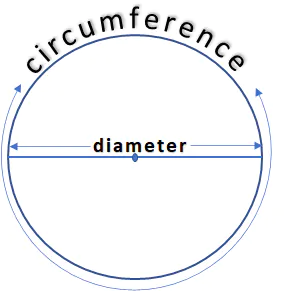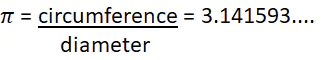Since PI continues into infinity, the greater the number of decimal places used when PI is used in an equation, the greater will be the degree of precision of the resulting calculation. Many people use 3.14 as the value of PI, especially when doing manual calculations, but Excel has the value of PI stored as a function accurate to 15 digits or 14 decimal places.

## The PI function in Excel

The PI function in Excel is found under the Math & Trig functions in Excel’s Function Library. It has no arguments, so it is written as follows:

``=PI()``

The PI formula returns the value of PI accurately to 14 decimal places, or 3.14159265358979. However, the output may be rounded to fewer decimal places depending on the number format applied to your output cell.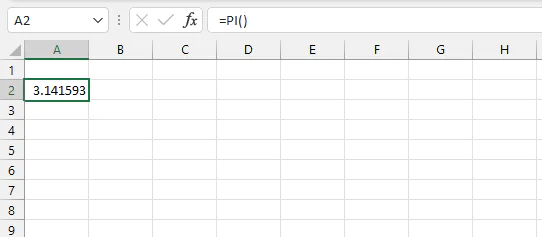If you need to see fewer or more decimal places, simply increase or decrease the rounding from the Number command group on the Excel ribbon. This is located on the Home tab.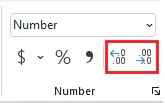Whether or not all 14 decimals are displayed, Excel will use all of them in its calculation, so the accuracy of the computation is not affected.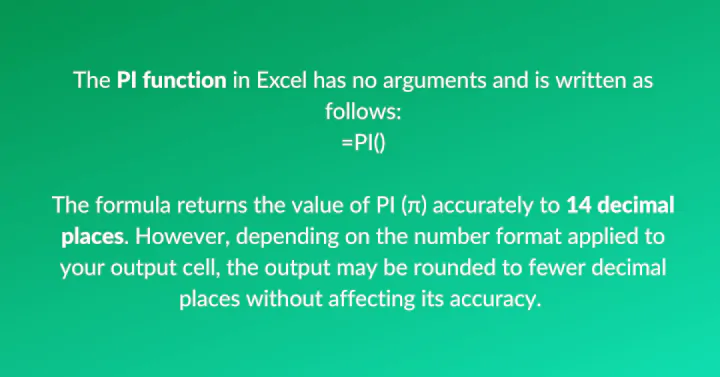## Using PI in Excel

In the following example, the PI function is used to calculate the volume of three cylinders, where the formula for volume is: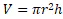In the above equation, r represents the radius or the half of a circle’s diameter, and h is the height of the cylinder. Values are multiplied to get the volume of a cylindrical object. We multiply by PI the same as we would use Excel to multiply by any other constant.

``=PI()*B2^2*C2``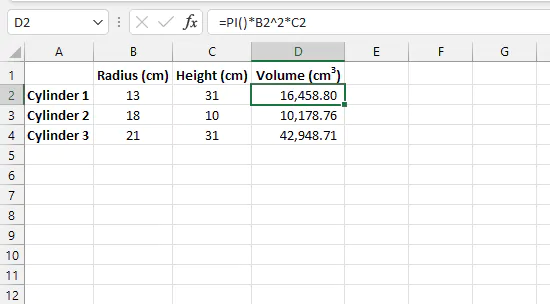In the above formula, we multiply each variable (squared radius and height) by PI, and the result will be the container’s volume in cubic units.

## PI in VBA

To use the PI constant in VBA, you can use the WorksheetFunction object. The PI function in VBA is

``Application.WorksheetFunction.PI()``

Otherwise, you can create a variable called “pi” and equate it to the worksheet PI function.

````pi = Application.WorksheetFunction.PI()`
```

Then you can use the variable “pi” in all the subsequent lines of VBA code for that function or subroutine.

If you store the variable as a Single, it only contains 7 digits.

When the variable is stored as a Double, it contains all 15 digits.

## PI symbol in Excel

If you want to insert the PI symbol in Excel, there are a few options.

### Option 1 - Use the Symbol font+P

This method is super-simple and may be the easiest to remember.

1. Change the font to Symbol
2. Type the letter P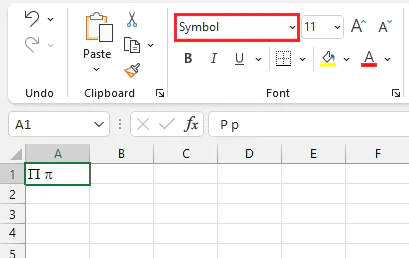An uppercase “P” will produce an uppercase PI symbol, and a lowercase “p” will give a lowercase PI.

### Option 2 - Use the Insert Symbol command

The PI symbol may also be inserted as a special symbol.

1. Find to the Insert tab on the Excel ribbon and click on Symbols
2. Click the Symbol command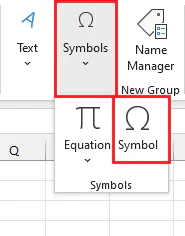3. From the Symbol window, select the Greek and Coptic subset
4. Look for the PI symbol, click Insert (or double-click) and Close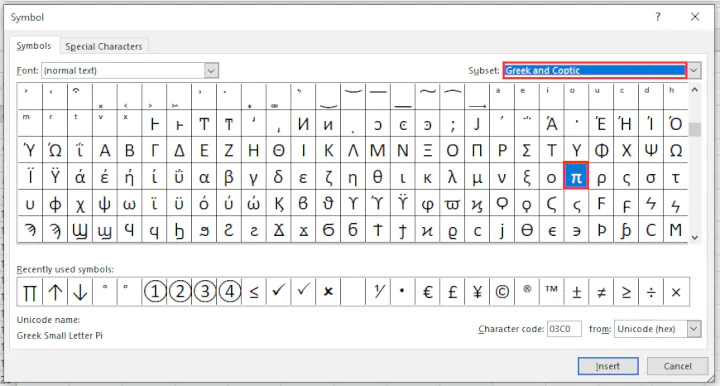The PI symbol will be inserted into the active cell. The font and size of the character may be changed from the Font command group as with any normal character.

### Option 3 - Use as a worksheet object

Mathematical symbols, including PI, can also be inserted as an object using the Insert Equation command from the Symbol command group.

1. Go to the Insert tab on the Excel ribbon and click on Symbols
2. Click the Equation command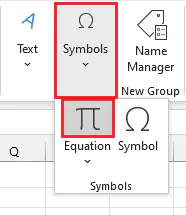3. Scroll through the available symbols and click on the PI symbol.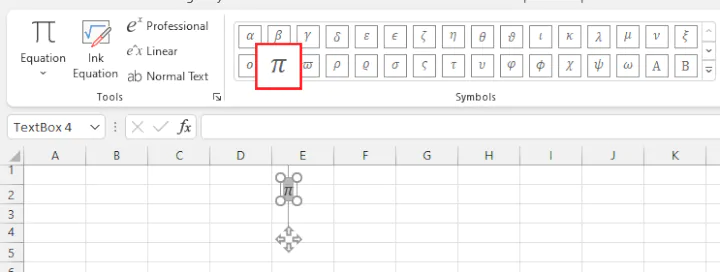The symbol will be inserted as a text element within an object in the Excel worksheet. The object can be moved around on the worksheet by dragging and dropping, as you would do with any other object.

Take your Excel skills to the next level with our comprehensive (and free) ebook!

## Key takeaways

Excel has many other built-in functions that simplify other mathematical calculations as well. GoSkills offers a variety of courses to help you learn the basics of Excel and its functions, along with other essential business skills. You can also learn to create charts and graphs to visualize data effectively.

• PI is a mathematical constant that represents the ratio of a circle's circumference to its diameter. It's a popular number in mathematics that pops up frequently in the physical world.
• In Excel, the PI function accurately calculates PI to 14 decimal places or more.
• The PI function in Excel is located in the Math & Trig functions in the Function Library.
• Excel uses all 14 decimal places when calculating with PI, regardless of whether they are displayed or not.
• The PI symbol can be inserted in Excel using the Symbol font, Insert Symbol command, or Insert Equation command.
• Excel offers other built-in functions for simplifying various mathematical calculations.

Become a certified Excel ninja with GoSkills bite-sized courses#### Join the Excel conversation on Slack

Ask a question or join the conversation for all things Excel on our Slack channel.Claudia Buckley

Claudia is a project manager and business skills instructor at GoSkills. In her spare time, she reads mystery novels and does genealogy research.